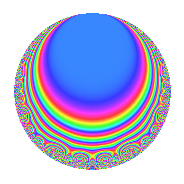Properties

 Label 22.3.dLevel 22 Weight 3 Character orbit d Rep. character $$\chi_{22}(7,\cdot)$$ Character field $$\Q(\zeta_{10})$$ Dimension 8 Newforms 1 Sturm bound 9 Trace bound 0

Related objects

Defining parameters

 Level: $$N$$ = $$22 = 2 \cdot 11$$ Weight: $$k$$ = $$3$$ Character orbit: $$[\chi]$$ = 22.d (of order $$10$$ and degree $$4$$) Character conductor: $$\operatorname{cond}(\chi)$$ = $$11$$ Character field: $$\Q(\zeta_{10})$$ Newforms: $$1$$ Sturm bound: $$9$$ Trace bound: $$0$$

Dimensions

The following table gives the dimensions of various subspaces of $$M_{3}(22, [\chi])$$.

Total New Old
Modular forms 32 8 24
Cusp forms 16 8 8
Eisenstein series 16 0 16

Trace form

 $$8q$$ $$\mathstrut -\mathstrut 2q^{3}$$ $$\mathstrut +\mathstrut 4q^{4}$$ $$\mathstrut +\mathstrut 2q^{5}$$ $$\mathstrut -\mathstrut 20q^{6}$$ $$\mathstrut -\mathstrut 30q^{7}$$ $$\mathstrut -\mathstrut 4q^{9}$$ $$\mathstrut +\mathstrut O(q^{10})$$ $$8q$$ $$\mathstrut -\mathstrut 2q^{3}$$ $$\mathstrut +\mathstrut 4q^{4}$$ $$\mathstrut +\mathstrut 2q^{5}$$ $$\mathstrut -\mathstrut 20q^{6}$$ $$\mathstrut -\mathstrut 30q^{7}$$ $$\mathstrut -\mathstrut 4q^{9}$$ $$\mathstrut -\mathstrut 4q^{11}$$ $$\mathstrut +\mathstrut 24q^{12}$$ $$\mathstrut +\mathstrut 30q^{13}$$ $$\mathstrut +\mathstrut 16q^{14}$$ $$\mathstrut +\mathstrut 42q^{15}$$ $$\mathstrut -\mathstrut 8q^{16}$$ $$\mathstrut +\mathstrut 30q^{17}$$ $$\mathstrut +\mathstrut 40q^{18}$$ $$\mathstrut -\mathstrut 30q^{19}$$ $$\mathstrut -\mathstrut 4q^{20}$$ $$\mathstrut +\mathstrut 24q^{22}$$ $$\mathstrut -\mathstrut 104q^{23}$$ $$\mathstrut -\mathstrut 40q^{24}$$ $$\mathstrut -\mathstrut 12q^{25}$$ $$\mathstrut -\mathstrut 96q^{26}$$ $$\mathstrut -\mathstrut 26q^{27}$$ $$\mathstrut -\mathstrut 40q^{28}$$ $$\mathstrut -\mathstrut 10q^{29}$$ $$\mathstrut -\mathstrut 60q^{30}$$ $$\mathstrut +\mathstrut 46q^{31}$$ $$\mathstrut -\mathstrut 14q^{33}$$ $$\mathstrut +\mathstrut 112q^{34}$$ $$\mathstrut +\mathstrut 70q^{35}$$ $$\mathstrut -\mathstrut 12q^{36}$$ $$\mathstrut +\mathstrut 6q^{37}$$ $$\mathstrut +\mathstrut 108q^{38}$$ $$\mathstrut +\mathstrut 130q^{39}$$ $$\mathstrut +\mathstrut 80q^{40}$$ $$\mathstrut +\mathstrut 250q^{41}$$ $$\mathstrut +\mathstrut 56q^{42}$$ $$\mathstrut -\mathstrut 12q^{44}$$ $$\mathstrut -\mathstrut 136q^{45}$$ $$\mathstrut -\mathstrut 160q^{46}$$ $$\mathstrut -\mathstrut 54q^{47}$$ $$\mathstrut -\mathstrut 8q^{48}$$ $$\mathstrut -\mathstrut 144q^{49}$$ $$\mathstrut -\mathstrut 80q^{50}$$ $$\mathstrut -\mathstrut 30q^{51}$$ $$\mathstrut -\mathstrut 40q^{52}$$ $$\mathstrut -\mathstrut 274q^{53}$$ $$\mathstrut -\mathstrut 26q^{55}$$ $$\mathstrut +\mathstrut 48q^{56}$$ $$\mathstrut -\mathstrut 130q^{57}$$ $$\mathstrut +\mathstrut 64q^{58}$$ $$\mathstrut +\mathstrut 50q^{59}$$ $$\mathstrut +\mathstrut 116q^{60}$$ $$\mathstrut +\mathstrut 50q^{61}$$ $$\mathstrut +\mathstrut 20q^{62}$$ $$\mathstrut -\mathstrut 20q^{63}$$ $$\mathstrut +\mathstrut 16q^{64}$$ $$\mathstrut -\mathstrut 136q^{66}$$ $$\mathstrut +\mathstrut 112q^{67}$$ $$\mathstrut +\mathstrut 60q^{68}$$ $$\mathstrut +\mathstrut 76q^{69}$$ $$\mathstrut +\mathstrut 4q^{70}$$ $$\mathstrut +\mathstrut 54q^{71}$$ $$\mathstrut -\mathstrut 80q^{72}$$ $$\mathstrut -\mathstrut 70q^{73}$$ $$\mathstrut -\mathstrut 40q^{74}$$ $$\mathstrut +\mathstrut 318q^{75}$$ $$\mathstrut +\mathstrut 266q^{77}$$ $$\mathstrut +\mathstrut 104q^{78}$$ $$\mathstrut +\mathstrut 370q^{79}$$ $$\mathstrut +\mathstrut 48q^{80}$$ $$\mathstrut +\mathstrut 180q^{81}$$ $$\mathstrut -\mathstrut 96q^{82}$$ $$\mathstrut -\mathstrut 150q^{83}$$ $$\mathstrut -\mathstrut 120q^{84}$$ $$\mathstrut -\mathstrut 330q^{85}$$ $$\mathstrut -\mathstrut 72q^{86}$$ $$\mathstrut +\mathstrut 72q^{88}$$ $$\mathstrut +\mathstrut 24q^{89}$$ $$\mathstrut +\mathstrut 160q^{90}$$ $$\mathstrut -\mathstrut 294q^{91}$$ $$\mathstrut -\mathstrut 112q^{92}$$ $$\mathstrut -\mathstrut 134q^{93}$$ $$\mathstrut -\mathstrut 20q^{94}$$ $$\mathstrut -\mathstrut 330q^{95}$$ $$\mathstrut -\mathstrut 18q^{97}$$ $$\mathstrut -\mathstrut 308q^{99}$$ $$\mathstrut +\mathstrut O(q^{100})$$

Decomposition of $$S_{3}^{\mathrm{new}}(22, [\chi])$$ into irreducible Hecke orbits

Label Dim. $$A$$ Field CM Traces $q$-expansion
$$a_2$$ $$a_3$$ $$a_5$$ $$a_7$$
22.3.d.a $$8$$ $$0.599$$ 8.0.64000000.1 None $$0$$ $$-2$$ $$2$$ $$-30$$ $$q+\beta _{1}q^{2}+(1-\beta _{1}-2\beta _{2}+\beta _{3}+2\beta _{4}+\cdots)q^{3}+\cdots$$

Decomposition of $$S_{3}^{\mathrm{old}}(22, [\chi])$$ into lower level spaces

$$S_{3}^{\mathrm{old}}(22, [\chi]) \cong$$ $$S_{3}^{\mathrm{new}}(11, [\chi])$$$$^{\oplus 2}$$